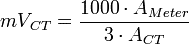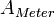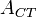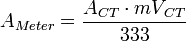The output voltage of a current transformer (CT) can be tested in the field using an digital multimeter with a millivolt AC (mVac) range. This test is useful to confirm that the CT is working properly and that current is flowing in the conductor that the CT is installed on.

If the current (amps) flowing in the conductor can be measured with a clamp on current meter or estimated from the connected load, this current value can be compared with the measured output voltage of the CT:$mV!_{CT}=frac{1000 cdot A_{Meter}}{3 cdot A_{CT}}$ AC millivolts measured on the CT outputs (between the white and black wires). The output voltage of the CT should be 333 mVac when the full-scale current rating of the CT is flowing in the conductor.$A_{Meter}$ Measured with clamp meter (or estimated) current through the CT.$A_{CT}$ Rated current of the CT.

You can use the CT output voltage to calculate the current (amps) flowing in the conductor:$A_{Meter}=frac {A_{CT} cdot mV!_{CT}}{333}$

Note: If the values you calculate do not seem correct, contact CCS technical support and we can check your numbers.